# 4. Complete the diagram below to show calcium and chloride ions being surrounded by water when CaCl2 dissolves and...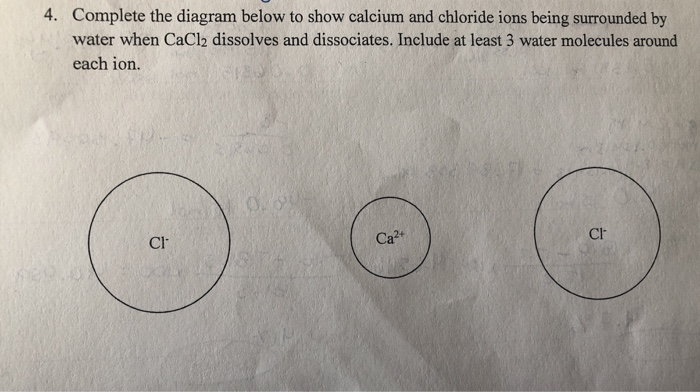4. Complete the diagram below to show calcium and chloride ions being surrounded by water when CaCl2 dissolves and dissociates. Include at least 3 water molecules around each ion. Ca2 Cl Cl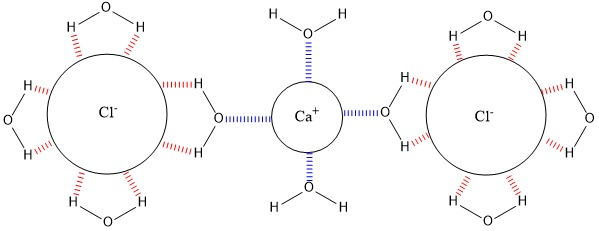In this image, the red color dashed lines are showing the interaction of the Hydrogen atom of the water molecule with the chloride ions negative charge while the interaction of the oxygen atom of the water molecule with the calcium ions positive charge in blue color. There will be many more water molecules surrounding both the type of ions which will cause the dissolve and dissociation of CaCl2 in water molecules.

##### Add Answer of: 4. Complete the diagram below to show calcium and chloride ions being surrounded by water when CaCl2 dissolves and...
Similar Homework Help Questions
• ### When an ionic compound dissolves in water, the cations and anions are separated. Ions in solution...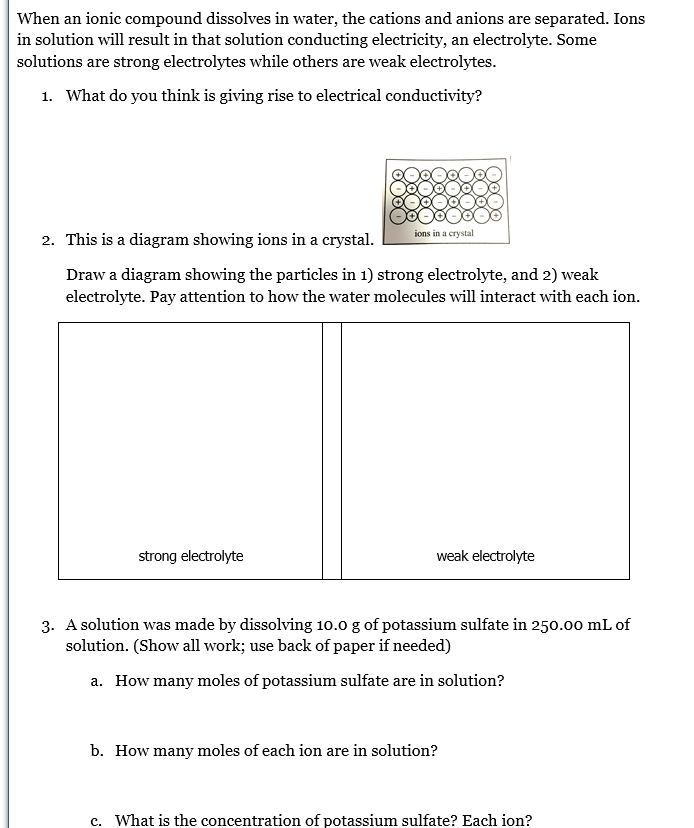When an ionic compound dissolves in water, the cations and anions are separated. Ions in solution will result in that solution conducting electricity, an electrolyte. Some solutions are strong electrolytes while others are weak electrolytes. 1. What do you think is giving rise to electrical conductivity? ions in a crystal 2. This is a diagram showing ions in a crystal. Draw a diagram showing the particles in 1) strong electrolyte, and 2) weak electrolyte. Pay attention to how the water...

• ### When calcium carbonate is added to hydrochloric acid, calcium chloride, carbon dioxide, and water are produc CaCO3(...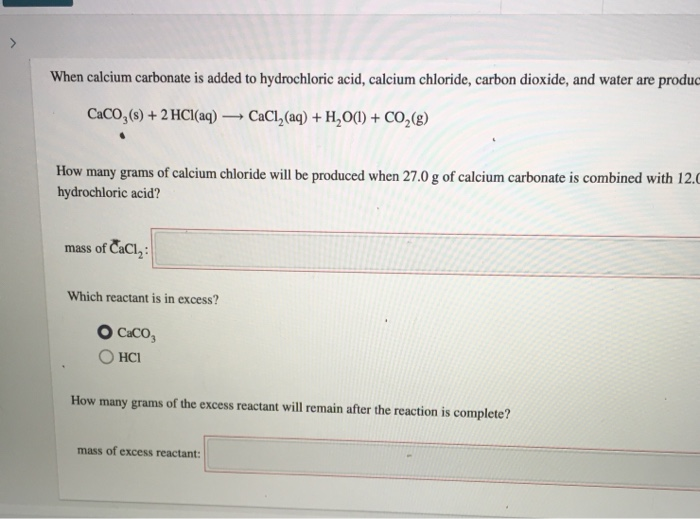When calcium carbonate is added to hydrochloric acid, calcium chloride, carbon dioxide, and water are produc CaCO3(s) + 2 HCl(aq) CaCl (aq) + H2O(l) + CO2(g) How many grams of calcium chloride will be produced when 27.0 g of calcium carbonate is combined with 12. hydrochloric acid? mass of CaCl2: Which reactant is in excess? O Caco, OHCI How many grams of the excess reactant will remain after the reaction is complete? mass of excess reactant:

• ### When calcium carbonate is added to hydrochloric acid, calcium chloride, carbon dioxide, and water are produced. CaCO3(s...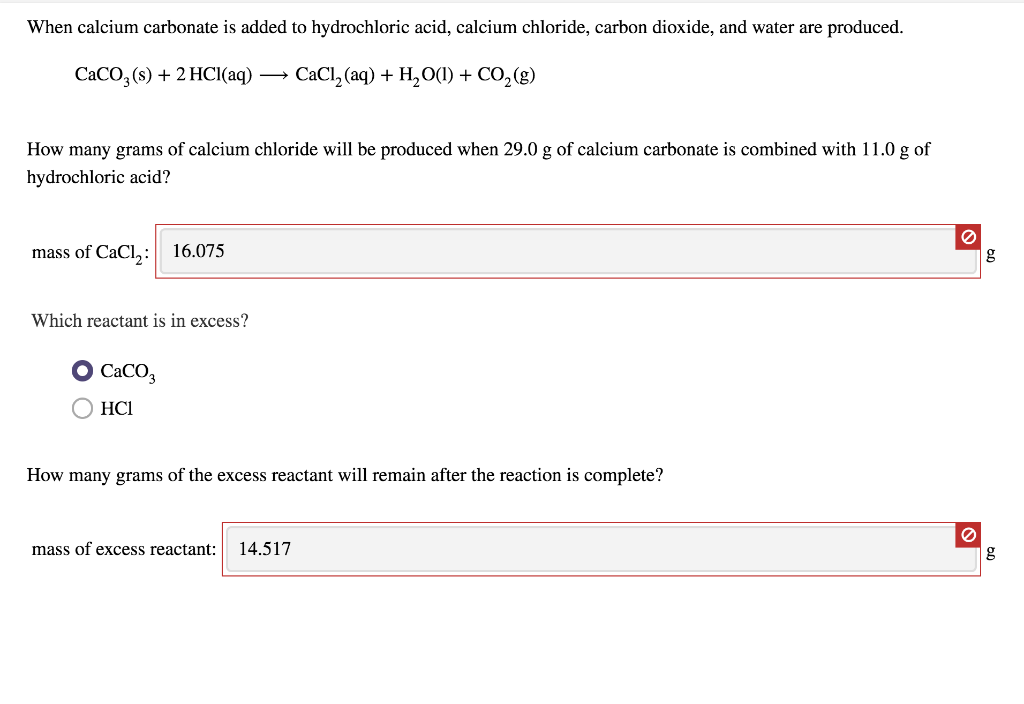When calcium carbonate is added to hydrochloric acid, calcium chloride, carbon dioxide, and water are produced. CaCO3(s) + 2 HCl(aq) → CaCl2 (aq) + H2O(1) + CO2(8) How many grams of calcium chloride will be produced when 29.0 g of calcium carbonate is combined with 11.0 g of hydrochloric acid? mass of CaCl,: 16.075 g Which reactant is in excess? Caco OHCI How many grams of the excess reactant will remain after the reaction is complete? mass of excess reactant:...

• ### Hard water often contains dissolved calcium ion and magnesium ions. One way to soften water is to add phosphate salts. T...

Hard water often contains dissolved calcium ion and magnesium ions. One way to soften water is to add phosphate salts. The phosphate ion forms insoluble precipitates with calcium and magnesium ions, removing them from solution. Suppose that a solution is 5.5×10−2 M in calcium chloride and 9.5×10−2 M in magnesium nitrate. What mass of sodium phosphate would have to be added to 11.5 L of this solution to completely eliminate the hard water ions? (Assume complete reaction.)

• ### 5) When KCl dissolves in water A) the Clions are attracted to dissolved K+ ions. B) the Clions are attracted to the...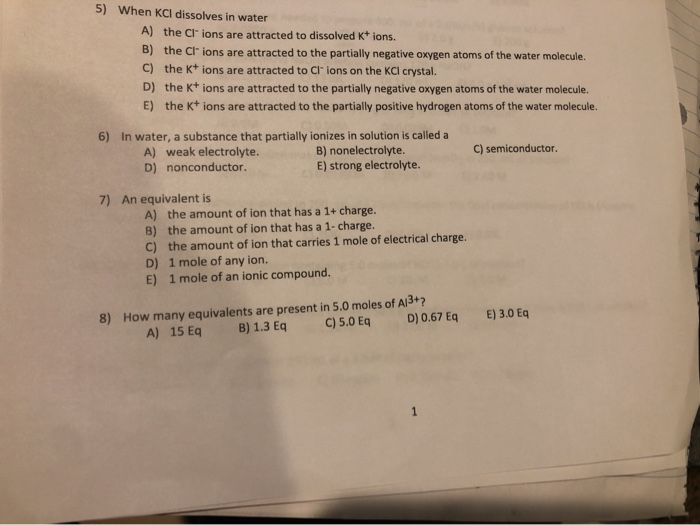5) When KCl dissolves in water A) the Clions are attracted to dissolved K+ ions. B) the Clions are attracted to the partially negative oxygen atoms of the water molecule. C) the K+ ions are attracted to Clions on the KCl crystal. D) the Kions are attracted to the partially negative oxygen atoms of the water molecule. E) the Kions are attracted to the partially positive hydrogen atoms of the water molecule. 6) in water, a substance that partially ionizes...

• ### 5. An unknown salt consists of two types of cations and one type of anion. The possible an- ions include chloride,...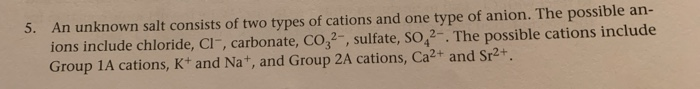5. An unknown salt consists of two types of cations and one type of anion. The possible an- ions include chloride, Cl, carbonate, CO32-, sulfate, SO 2-. The possible cations include Group 1A cations, K+ and Nat, and Group 2A cations, Ca2+ and Sr2+. a) After dissolving the unknown in 20 mL of DI water, you add 0.5 mL of 0.05 M AgNO3 and observe a precipitate. What are the possible precipitates that are formed? Write the net ionic equations...

• ### 3. 10 points. Calculate the amounts (kg, 100% basis) of H2SO4 and NaOH required in a dual bed ion exchange system to re...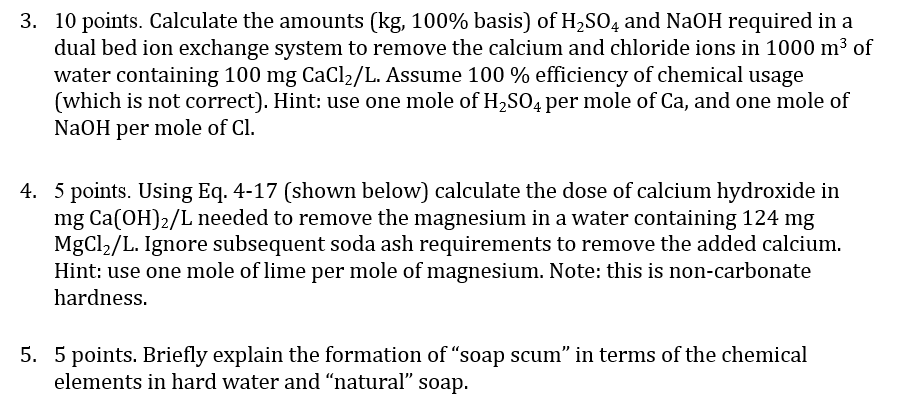3. 10 points. Calculate the amounts (kg, 100% basis) of H2SO4 and NaOH required in a dual bed ion exchange system to remove the calcium and chloride ions in 1000 m3 of water containing 100 mg CaCl2/L. Assume 100 % efficiency of chemical usage (which is not correct). Hint: use one mole of H2SO4 per mole of Ca, and one mole of NaOH per mole of Cl 4. 5 points. Using Eq. 4-17 (shown below) calculate the dose of calcium...

• ### Lab Section: 1 Prelab: Inorganic Group I Ions 1. On the report sheet, complete the flow...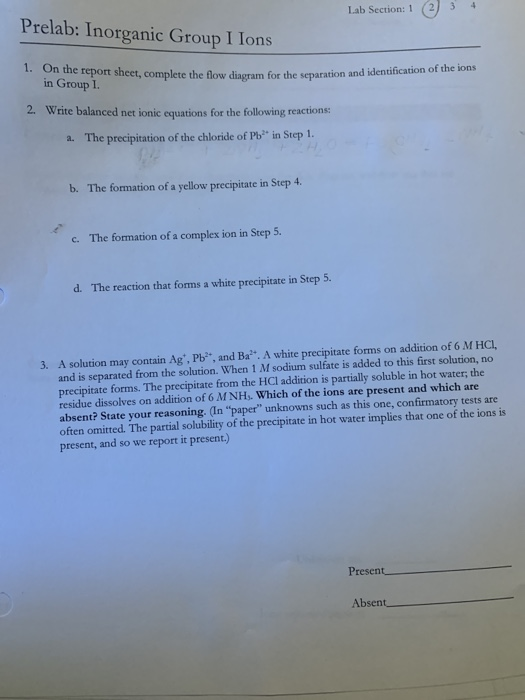Lab Section: 1 Prelab: Inorganic Group I Ions 1. On the report sheet, complete the flow diagram for the separation and identification of the ions in Group I 2. Write balanced net ionic equations for the following reactions: The precipitation of the chloride of Pb a. in Step 1. The formation of a yellow precipitate in Step 4. b. The formation of a complex ion in Step 5. c. d. The reaction that forms a white precipitate in Step 5....

• ### A small amount of sodium bromide (NaBr) is dissolved in a large amount of water. Imagine separating this process into the four stages sketched below (Tes sketches show only a portion of the subst...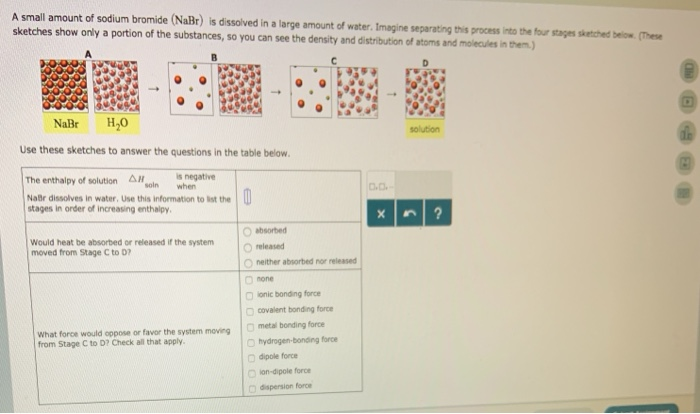A small amount of sodium bromide (NaBr) is dissolved in a large amount of water. Imagine separating this process into the four stages sketched below (Tes sketches show only a portion of the substances,so you can see the density and distribution of atoms and molecules in them) NaBr H20 solution Use these sketches to answer the questions in the table below The enthalpy of solution ln when is negative Naßr dissolves in water, Use this information to list the stages...

• ### Data analysis: write the net ionic equations for any reactions that occurred between the metals and metal ions list occurred with N.R. Indicate any combinations where no reaction ed below (sect...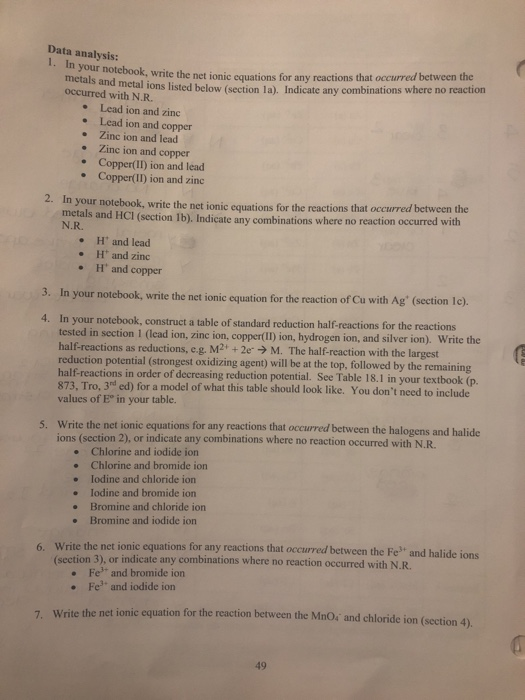Data analysis: write the net ionic equations for any reactions that occurred between the metals and metal ions list occurred with N.R. Indicate any combinations where no reaction ed below (section la). e Lead ion and zinc . Lead ion and copper Zinc ion and lead . Zinc ion and copper . Copper(II) ion and lead . Copper(II) ion and zinc 2. In your notebook, write the net ionic equations for the react tions that occurred between the metals and...

Need Online Homework Help?